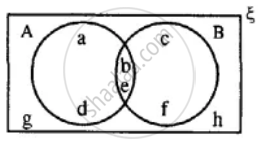# Using the Given Diagram, Express the Following Sets in the Terms of a and B. - Mathematics

Diagram

Using the given diagram, express the following sets in the terms of A and B. {g, h}#### Solution

{g, h} = (A ∪ B)'

[∵ A ∪ B = {a, b, c, d, e, f} ∴ (A ∪ B)' = {g, h}]

Concept: Venn Diagram
Is there an error in this question or solution?

#### APPEARS IN

Selina Concise Mathematics Class 8 ICSE
Chapter 6 Sets
Exercise 6 (E) | Q 11.5 | Page 76